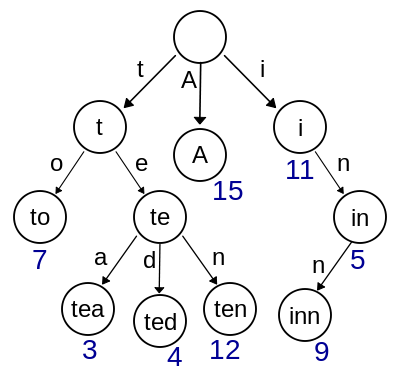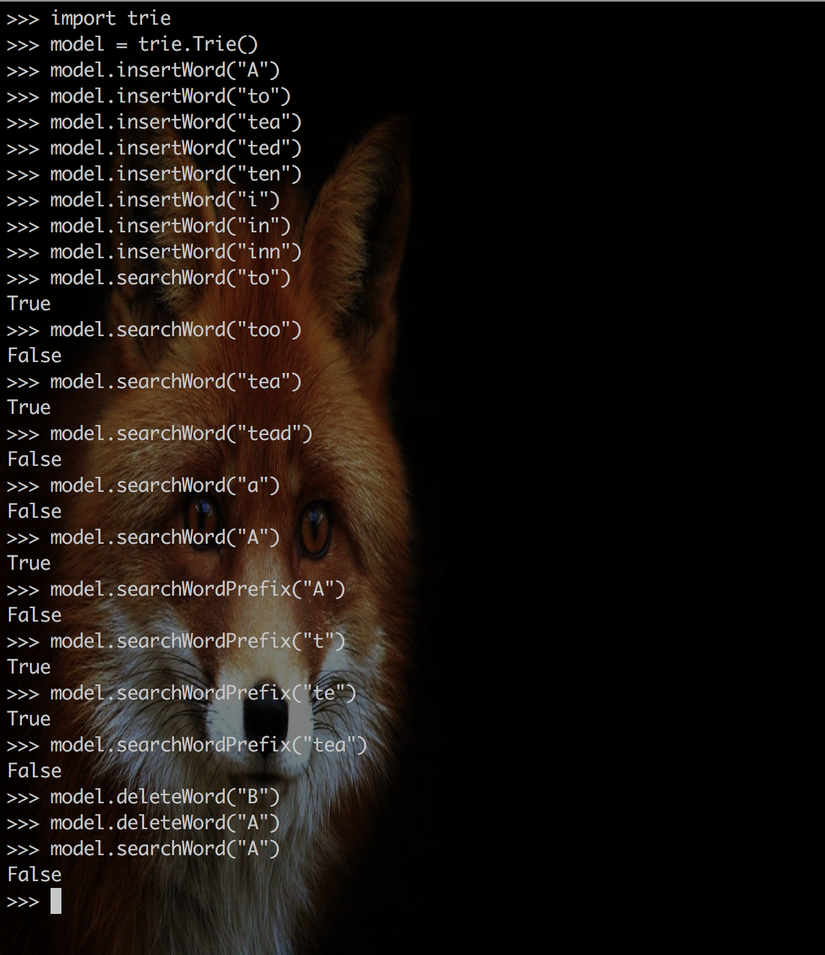+1

# NLP: Build a Trie Data structure from scratch with pythonA trie is a tree-like data structure whose nodes store the letters of an alphabet. By structuring the nodes in a particular way, words and strings can be retrieved from the structure by traversing down a branch path of the tree. The common usage of tries can be found in for autocomplete features, like the one used in search engines like Google. Moreover, Trie can also be usefull for developing NLP model because a computer does know human language. Therefore, we need to separate a sentence into words then encode them so we can fit it into our NLP model. This article can help you learn more about trie.

### Algorithms

We are going to write an algorithms to add, find and delete Trie node. Firstly, we define the `trie` class and init root node.

``````# Trie class
class Trie:
# init Trie class
def __init__(self):
self.root = {"isEndOfWord": False, "children": {}}
``````

*Note: trie node use python datatype `dict` because it help us to save/load file with ease.

Adding word into node can proceed by looping through each character of the string to be inserted, then it append new nodes for the suffix of the string that is not contained in the tree:

``````  def insertWord(self, word):
current = self.root
for ch in word:

if current["children"].has_key(ch):
node = current["children"][ch]
else:
node = self.getNode()
current["children"][ch] = node

current = node
current["isEndOfWord"] = True
``````

To find `word` which contain in trie or not, we loop through each character of the string then we truen false if node doesn't contain each charater of the string or th node for the last charater still has children. otherwise, we return true.

``````  def searchWord(self, word):
current = self.root
for ch in word:
if not current["children"].has_key(ch):
return False
node = current["children"][ch]

current = node
return current["isEndOfWord"]
``````

We can also search the prefix of given word by semply check the node of last charater in word and return `true` if it has children.

``````  def searchWordPrefix(self, word):
current = self.root
for ch in word:
if not current["children"].has_key(ch):
return False
node = current["children"][ch]

current = node
# return True if children contain keys and values
return bool(current["children"])
``````

To remove node of word from trie, we just set the value `isEndOfWord` to `false`.

``````  def deleteWord(self, word):
self._delete(self.root, word, 0)

def _delete(self, current, word, index):
if(index == len(word)):
if not current["isEndOfWord"]:
return False
current["isEndOfWord"] = False
return len(current["children"].keys()) == 0

ch = word[index]
if not current["children"].has_key(ch):
return False
node = current["children"][ch]

should_delete_current_node = self._delete(node, word, index + 1)

if should_delete_current_node:
current["children"].pop(ch)
return len(current["children"].keys()) == 0

return False
``````

Finally, let put it together:

``````import pickle
import json
# Trie class
class Trie:
# init Trie class
def __init__(self):
self.root = self.getNode()

def getNode(self):
return {"isEndOfWord": False, "children": {}}

def insertWord(self, word):
current = self.root
for ch in word:

if current["children"].has_key(ch):
node = current["children"][ch]
else:
node = self.getNode()
current["children"][ch] = node

current = node
current["isEndOfWord"] = True

def searchWord(self, word):
current = self.root
for ch in word:
if not current["children"].has_key(ch):
return False
node = current["children"][ch]

current = node
return current["isEndOfWord"]

def searchWordPrefix(self, word):
current = self.root
for ch in word:
if not current["children"].has_key(ch):
return False
node = current["children"][ch]

current = node
# return True if children contain keys and values
return bool(current["children"])

def deleteWord(self, word):
self._delete(self.root, word, 0)

def _delete(self, current, word, index):
if(index == len(word)):
if not current["isEndOfWord"]:
return False
current["isEndOfWord"] = False
return len(current["children"].keys()) == 0

ch = word[index]
if not current["children"].has_key(ch):
return False
node = current["children"][ch]

should_delete_current_node = self._delete(node, word, index + 1)

if should_delete_current_node:
current["children"].pop(ch)
return len(current["children"].keys()) == 0

return False

def save_to_pickle(self, file_name):
f = open(file_name + ".pkl", "wb")
pickle.dump(self.root, f)
f.close()

f = open(file_name + ".pkl", "rb")
f.close()

def save_to_json(self, file_name):
json_data = json.dumps(self.root)
f = open(file_name + ".json", "w")
f.write(json_data)
f.close()

json_file = open(file_name + ".json", "r")
json_file.close()
``````

### TestingYes, it works.

### What's next?

Trie is one of the most powerful data structure to work with text where you can use it to build your own auto-suggestion, word checking, and more. Moreover, we can use it to prepare our text data for our NLP in the upcoming article.

Stay tune.